# RFBFN: A Relation - First Blank Filling Network for Joint Relational Triple Extraction

## Basic Idea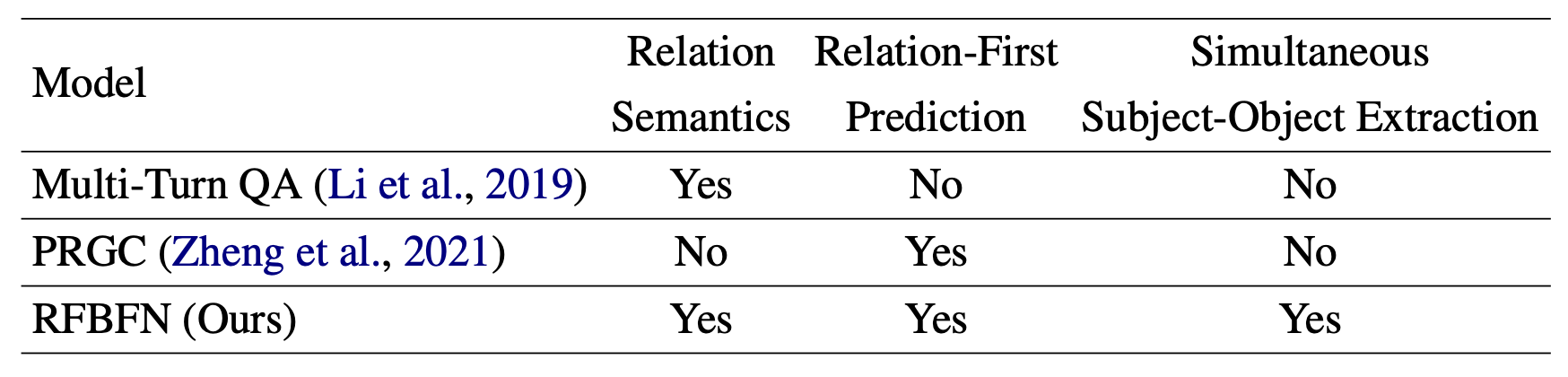## RFBFN

### Overview

1. Span - Level Encoder: 从输入文本中抽取出Span表示.
2. Relation Detection Module: 预测句子中的潜在关系, 并过滤掉不相关的关系.
3. Blank Filling Module: 用很多指明关系的模板作为输入, 预测该关系下对应的实体对.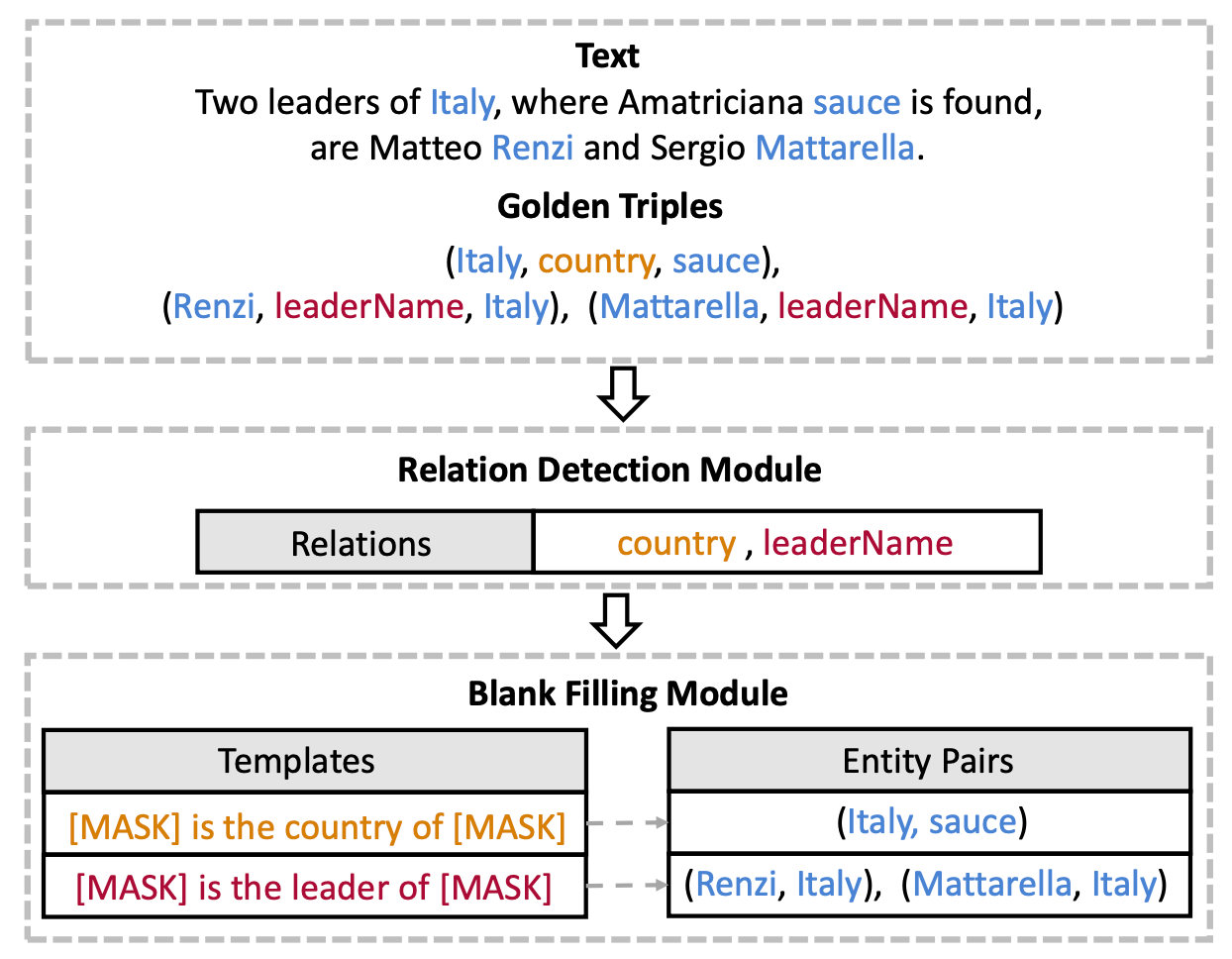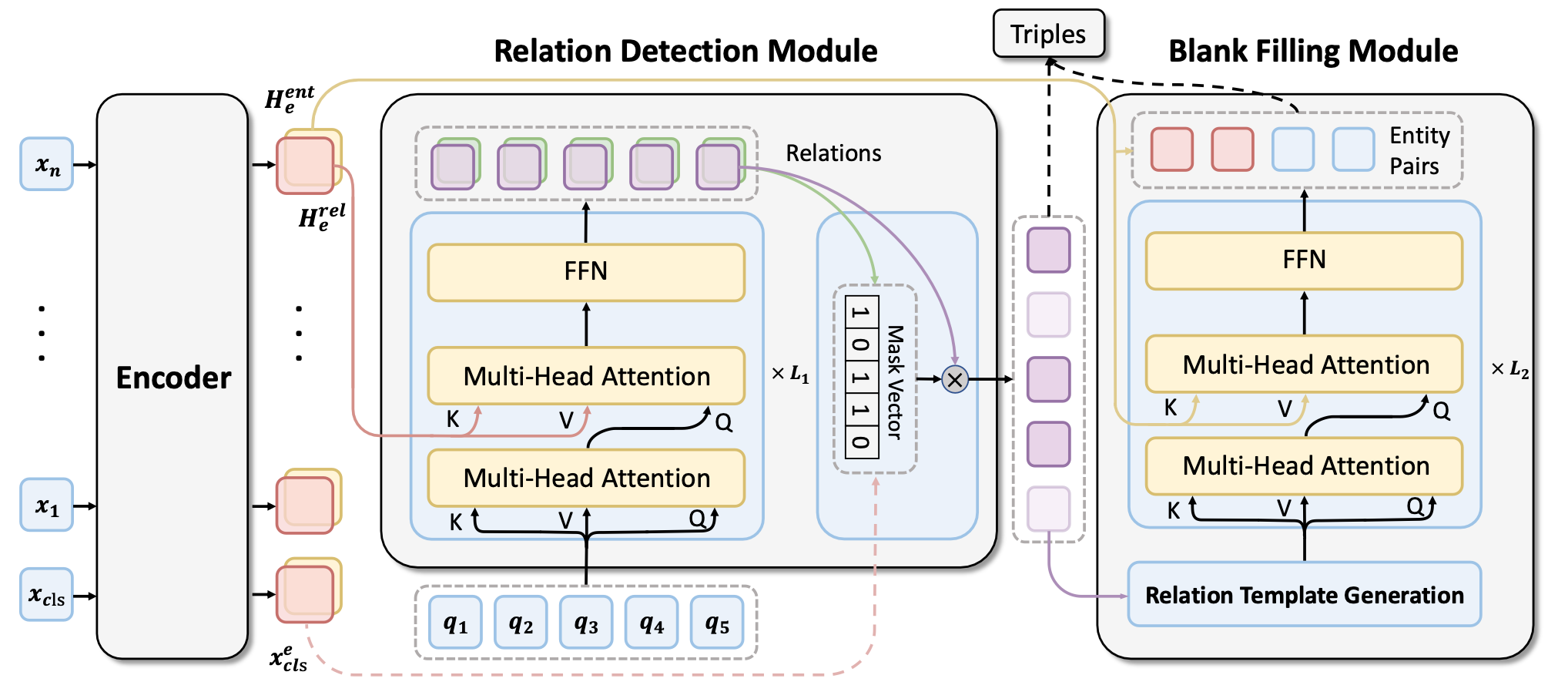### Span - Level Encoder

Span - Level Encoder通过BERT来抽取句子中的Span表示. 令$S=(s_1, s_2, \dots, s_{n_s})$ 为输入句子$X$ 中的所有Span, 则对于Span $s_i \in S$, 其表示$\mathbf{h}_i^e$ 定义为:

$$\mathbf{h}_{\mathrm{i}}^{\mathrm{e}}=\left[\mathbf{x}_{\mathrm{START}(\mathrm{i})}^{\mathrm{e}} ; \mathbf{x}_{\operatorname{END}(\mathrm{i})}^{\mathrm{e}} ; \phi\left(\mathbf{x}_{\mathrm{i}}\right)\right]$$

\begin{aligned} &\mathbf{H}_{\mathrm{e}}^{\mathrm{rel}}=\mathbf{W}_{r e l} \mathbf{H}^{e}+\mathbf{b}_{r e l} \\ &\mathbf{H}_{\mathrm{e}}^{\mathrm{ent}}=\mathbf{W}_{e n t} \mathbf{H}^{e}+\mathbf{b}_{e n t} \end{aligned}

### Relation Detection Module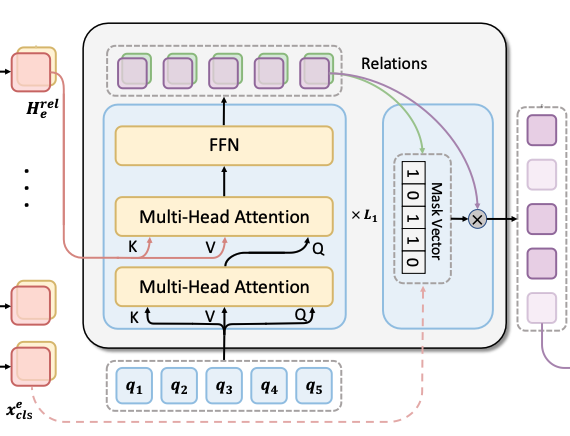#### Potential Relation Extractor

$$\mathbf{p}_{\mathrm{i}}^{\mathrm{r}}=\operatorname{Softmax}\left(\mathbf{W}_{\mathrm{r}} \mathbf{h}_{\mathrm{i}}^{\mathrm{r}}+\mathbf{b}_{\mathrm{r}}\right)$$

#### Candidate Relation Judgement

Candidate Relation Judgement以NAD的输出表征$\mathbf{H}^\mathrm{r}$ 和[CLS] 的Embedding作为输入, 以一个Mask向量$\mathbf{M}$ (其中的值都是布尔值)作为输出, 以确定该类型关系是否在该句子中成立:

$$\mathbf{M}=\sigma\left(\mathbf{W}_{\mathrm{s}}\left[\mathbf{H}^{\mathrm{r}} ; \mathbf{x}_{\mathrm{cls}}^{\mathrm{e}}\right]+\mathbf{b}_{\mathrm{s}}\right)$$

### Blank Filling Module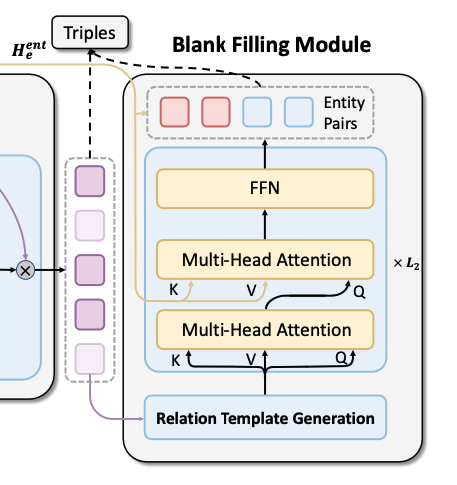#### Relation Template Generation

$$T_{r}=\left(m_{1}^{r}, t_{1}^{r}, t_{2}^{r}, \ldots, t_{n_{t}}^{r}, m_{2}^{r}\right)$$

#### Entity Pair Extractor

$$\mathbf{h}_{\mathrm{i}, \mathrm{r}}^{\mathrm{b}}=\tanh \left(\mathbf{W}_{\mathrm{b}}^{1} \bar{\mathbf{H}}+\mathbf{W}_{\mathrm{b}}^{2} \mathbf{h}_{\mathrm{i}, \mathrm{r}}^{\mathrm{blk}}+\mathbf{b}_{\mathrm{b}}\right)$$

$$\mathbf{p}_{\mathrm{i}, \mathrm{r}}^{\mathrm{b}}=\operatorname{Softmax}\left(\mathbf{u}_{\mathrm{b}}^{\mathrm{T}} \cdot \mathbf{h}_{\mathrm{i}, \mathrm{r}}^{\mathrm{b}}\right)$$

### Joint Training

$$\mathcal{L}_{e n t}=-\sum_{r=1}^{n_{d}} \sum_{i=1}^{2 k} \log \mathbf{p}_{\mathrm{i}, \mathrm{r}}^{\mathrm{b}}\left(\mathrm{y}_{\mathrm{i}, \mathrm{r}}^{\mathrm{b}}\right)$$

$$\pi^{\ast}=\underset{\pi \in \Pi\left(\mathrm{n}_{\mathrm{q}}\right)}{\operatorname{argmin}}\left(-\sum_{\mathrm{i}=1}^{\mathrm{I}_{\mathrm{q}}} \mathrm{I}\left(\mathrm{y}_{\mathrm{i}}^{\mathrm{r}}\right) \cdot \mathbf{p}_{\pi(\mathrm{i})}^{\mathrm{r}}\left(\mathrm{y}_{\mathrm{i}}^{\mathrm{r}}\right)\right)$$

$$\mathcal{L}_{r e l}=-\sum_{\mathrm{i}=1}^{\mathrm{n}_{\mathrm{q}}} \log \mathbf{p}_{\pi^{*}(\mathrm{i})}^{\mathrm{r}}\left(\mathrm{y}_{\mathrm{i}}^{\mathrm{r}}\right)$$

$$\mathcal{L}=\lambda \mathcal{L}_{e n t}+(1-\lambda) \mathcal{L}_{r e l}$$

## Experiments

### Datasets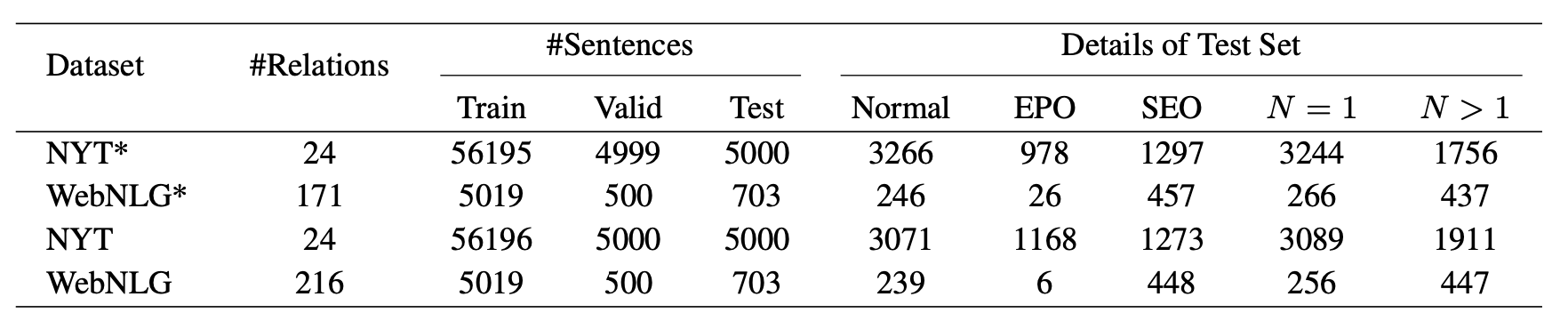### Main Results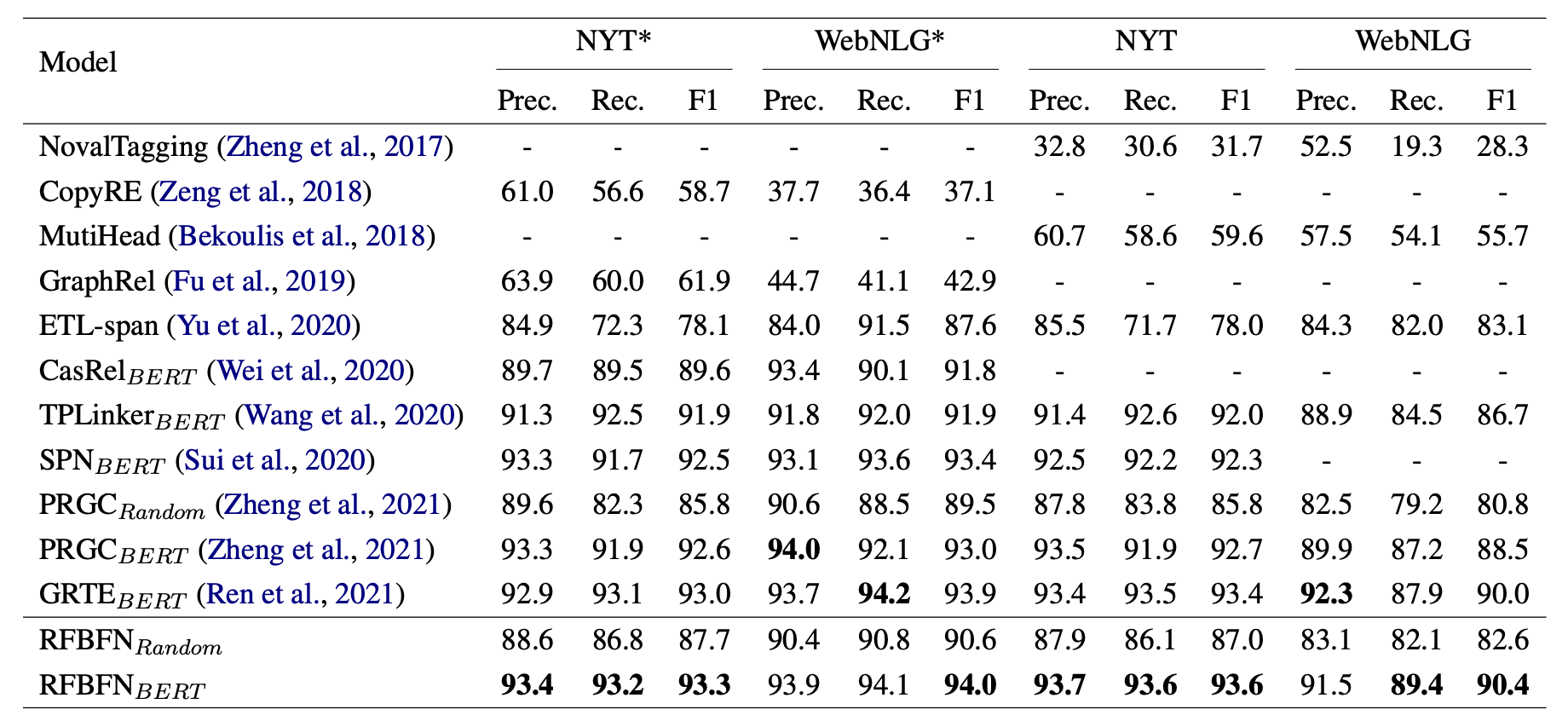### Detailed Results on Complex Scenarios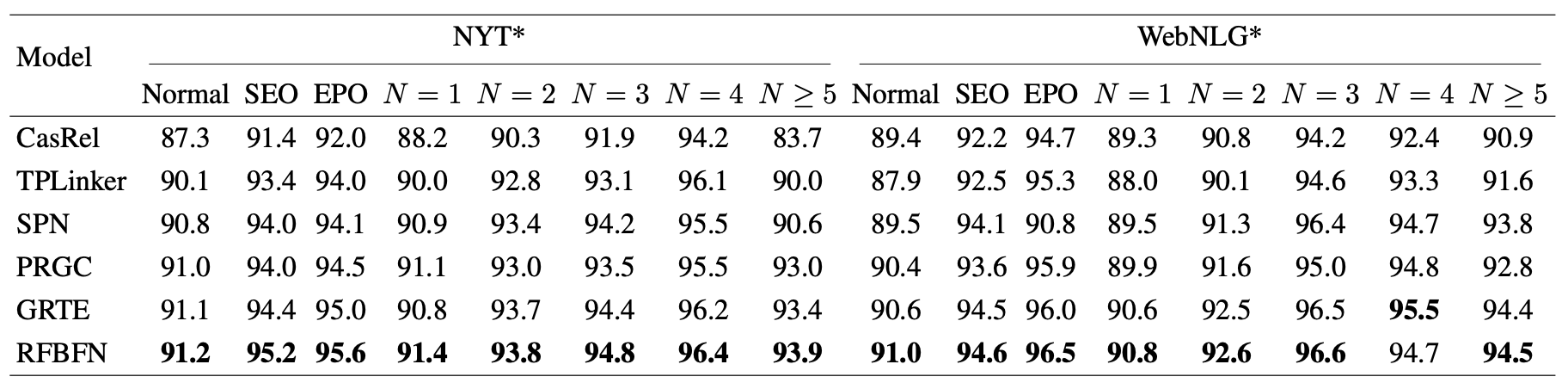RFBFN几乎全面优于Baseline模型.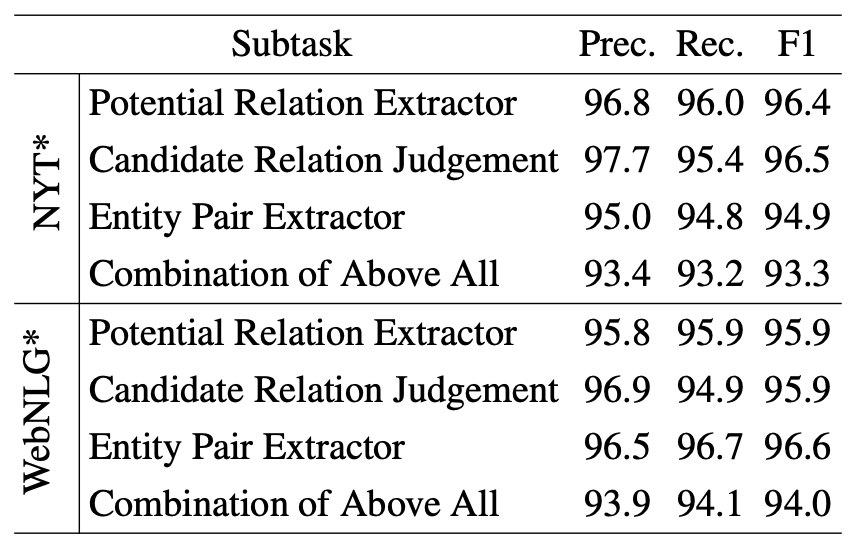### Analysis

#### Ablation Study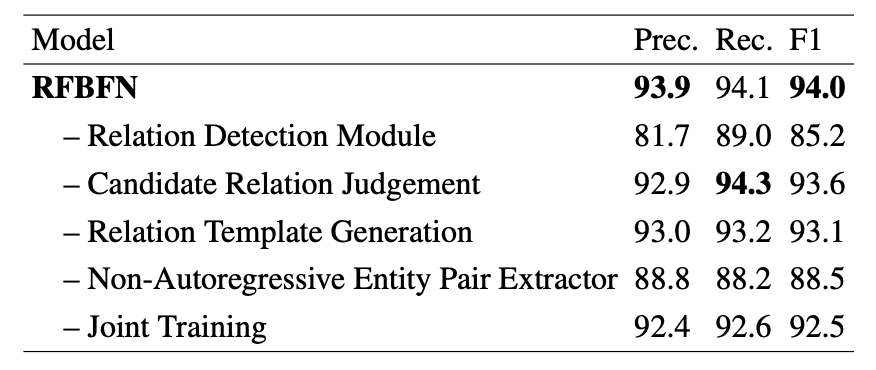• - Relation Detection Module: 直接移除Relation Detection Module, 假设句子中所有关系都成立, 喂给Entity Pair Extractor. 因为WebNLG* 关系太多了, 作者只选了正样本和30%的负样本.
• - Candidate Relation Judgement: 直接移除Candidate Relation Judgement, 即忽略Relation Detection Module里面抽取出的错误关系.
• - Relation Template Generation: 把Relation Template换成Relation Embedding.
• - Joint Training: 不再共享Encoder, 使用两个独立的Encoder做训练.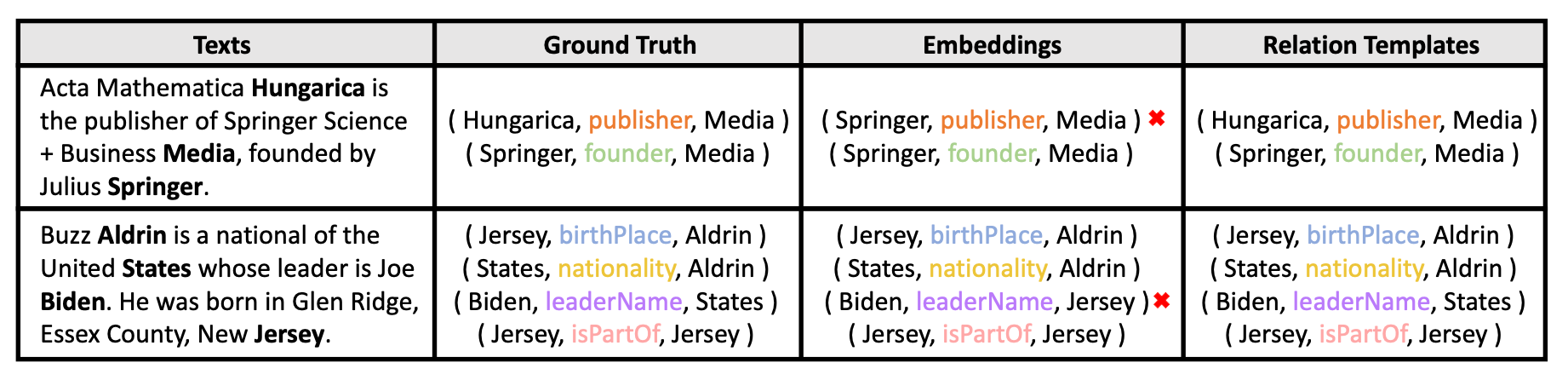#### Visualization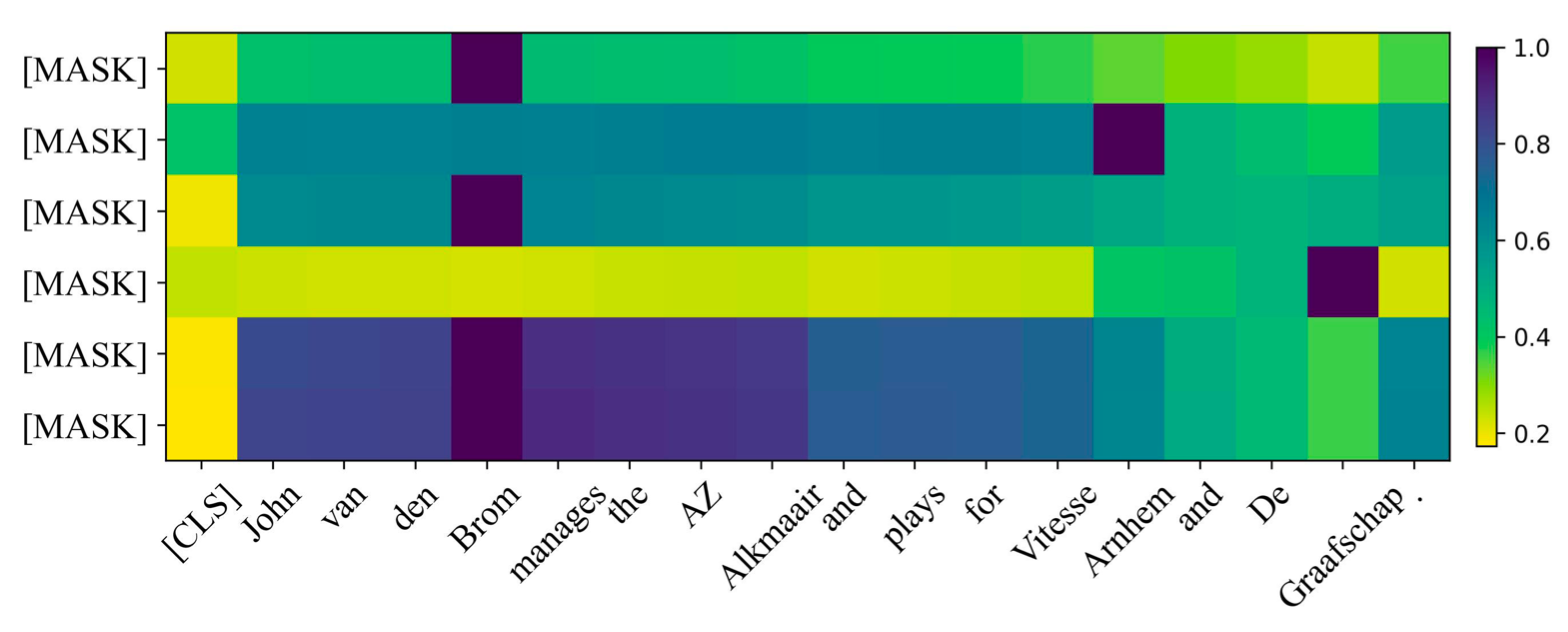## Summary

上一篇Pytorch学习: Pytorch Lightning
Pytorch学习: Pytorch LightningPytorch Lightning是在Pytorch基础上封装的框架, 号称”Pytorch里的Keras”, 如官网所述, 它具有灵活, 解耦, 易于复现, 自动化, 扩展性好等优点
2022-08-14SPN: Joint Entity and Relation Extraction with Set Prediction Networks
Joint Entity and Relation Extraction with Set Prediction Networks本文是论文Joint Entity and Relation Extraction with Set Pred
2022-06-22
目录